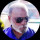Coffee Room
Discuss anything here - everything that you wish to discuss with fellow engineers.
12915 Members
Join this group to post and comment.mujju433 • Sep 30, 2008

# Why the power is in KVA?

Why the power is in KVA ? whats the reason? any reason?

What is the difference between relay and switch?Hi Mujju!
Power is measured in 2 ways- Watts and VA.
Both are not the same!!!
1. KVA represents work done in a DC circuit.
2. Watt is the measure of the total work done in an AC circuit.
Hence, Watt=VA x PF (Power Factor)
Or KW=KVAxPFFahad Bhatti • Oct 1, 2008
As far as my knowledge goes, switch is the generic name which may refer to a semiconductor device (transistor for example) while Relay is the special case of switch whose gate is magnetically insulated, its operating voltage and current is higher and is suitable for high power controls (usually higher than 10 amps).
In practice we use analog switch to reduce the power dissipation of relay (if required so).Farhad,relay can be also used for low-power applications its basic function is too convert electrical signals into mechanical one,and docel have rightly ans the question....its the best suited ansFahad Bhatti • Oct 1, 2008
oops, that's what i wrote in my last exam, thanks anyway sauravmujju433 • Oct 2, 2008
Hey docel can u elaborate more on KVA vs Watts?Re: Why the power is in KVA? Part-1

Hi Mujju!!

Ok- it is a little difficult without a complete understanding of the mutual relationship between Voltage, Resistance and Current.

1. Ohms Law states that a given Voltage across a given Resistance will result in a quantity of current of value I=V/R.
The Current I is proportional to and in step (Phase) with the applied Voltage.
This means that Vmin=Imin; Vmax=Imax. The current will increase simultaneously with the Voltage, with the Resistance being constant. This is a pure DC circuit.

2. In an AC circuit, the Voltage and current are always changing periodically. If the Resistance is constant, then the Current is in step with the applied Voltage.

3. If the resistance varies in the above 2 DC circuits, certain funny things happen. It happens in the following conditions:

a) If the load is a Capacitance, the DC current flows for a very short period and stops after some finite time. The Current flow is not linear. It is maximum at the time of switch-on and exponentially reduces to zero. The voltage will appear to be 0 and will rise slowly in time. That is , the Current leads the Voltage. Here, the Voltage lags behind the Current. The capacitance initially acts like a short-circuit and gradually becomes an open circuit.
b) If the Load is an inductor, even more funny things happen. At the time of switch-on, the DC Voltage is maximum and the DC current is minimum. The Current will gradually rise after some time and is out of step ( out of Phase) with the applied DC voltage. That is, the Current lags behind the voltage. The Inductance appears as a high resistance (open circuit) and gradually reduces to a low resistance (short circuit).

In the above 2 conditions, the load acts as a variable resistance. The Capacitor resists any change in the voltage and the inductor opposes any change in the current. This becomes even more complex with the resistance of the wire etc., in the circuit.

The entire process goes crazy when you apply AC to the above circuits !

To be continued.......sriramchandrk • Oct 16, 2008
1st Question: Why power is in KVA.

We know that

P = VI
Where P = power(or work done)
v = voltage
I = current
Since unit for voltage is Volt and Unit for Current is Ampere
Power is read as VoltAmpere(or VA)

1000Volt-Ampere is called as 1KVA.

2nd Question: What is relay vs switch?
According to my understanding

Relay is used when you want to contol any electrical equipment using small DC voltage, eg: a small timer device can switch on/off street lights.
(Magnetism through electical induction is used for closing the circuit)

Switch is a mechanical device which can be used to control electrical equipments.

Thanks & Regards
Sriramkombo • Nov 20, 2008
Hi Mujju

I Agree with sriram about Kva.
As I Know 😁
Relay is device to make open and close an electrical circuit and controled by Electric/Ac or DC and
Switch have fuction like relay but controlled by mechanical process For example Limit Switch etc